Next: Expectation of the description Up: Computing the expectation of Previous: Computing the expectation of

## Feedforward equations

We shall consider a standard MLP-network with input, hidden and output layers. In order to be able to write the feedforward equations in a compact form, we shall assign all parameters and outputs of the neurons a unique index. In our notation,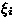can mean either the value of a parameter or output of a neuron, that is,are used to denote any value than can be an input for neurons. The set of indices for the parameters is denoted by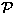and the transfer functions of the neurons are denoted by fi. The valuesare defined by equation 4.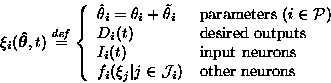(4)
Input and output data is parametrised by time t. We have used a shorthand notation for the parameters of a function. For example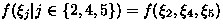. The set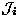thus contains the indices of the inputs to the neuron i or, equivalently, indices of the parameters of the function fi. The network is assumed to be strictly feedforward, which means that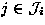implies j < i.

For hidden and output neurons the transfer functions fi are like in any conventional neural network. They can be sums of inputs multiplied by weights, sigmoids, radial basis functions, etc.

The cost function for supervised learning is L(MS) + L(DS | MS) as explained in section 1.1. The description lengths are computed according to equation 1, with the exception that the terms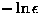are omitted from L(DS | MS). For each Di we shall assign a function fj, which is used to compute the terms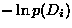. The set of indices for these functions is denoted by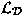. Similarly, the set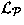comprises of the indices of functions fj, which evaluate the terms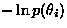. We can now write down the cost function in terms ofand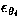.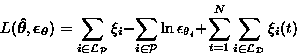(5)
The first two terms correspond to L(MS) and the third term to L(DS | MS).

If a parameter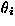does not have an associated neuron in the set, it means that we tacitly assume the probability distribution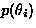to be constant throughout the range of values of, that is, we assume the value of the parameter to be evenly distributed. It has to be reminded that although the constant termcan be omitted when adapting the parameters and their accuracies, it should still be taken into account when models with different parametrisations are compared.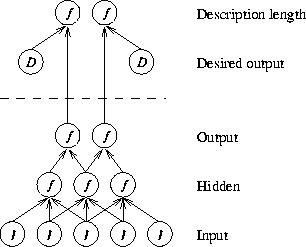The structure of the network is shown in figure 2. Desired outputs are marked by D, input neurons by I, and other neurons by f. The parameters of the network are not shown. The functions f above the dotted line are the ones used to compute the description length of the parameters and the data.Next: Expectation of the description Up: Computing the expectation of Previous: Computing the expectation of
Harri Lappalainen
5/19/1998